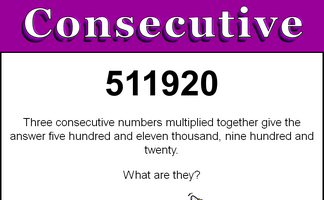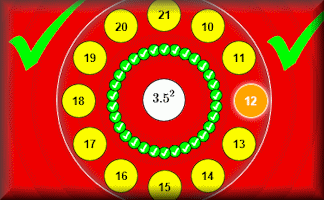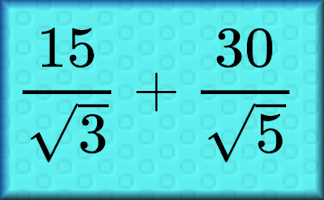#There are 366 different Starters of The Day, many to choose from. You will find below some starters on the topic of Roots. A lesson starter does not have to be on the same topic as the main part of the lesson or the topic of the previous lesson. It is often very useful to revise or explore other concepts by using a starter based on a totally different area of Mathematics.

Main Page

### Roots Starters:Three consecutive numbers multiplied together give a given product. Pupils are asked to figure out what the numbers are.Which three consecutive numbers multiplied together give the given answer.

## Exercises#### Bidmaze

Find your way through the maze encountering mathematical operations in the correct order to achieve the given total.#### Estimate Powers and Roots

Estimate the powers and roots of the given positive numbers.#### Square and Cube Roots

Find square roots and cube roots by first calculating the prime factorisation of a number.#### Surds

A self-marking exercise on calculating, simplifying and manipulating surds (also known as radicals).

### Search

The activity you are looking for may have been classified in a different way from the way you were expecting. You can search the whole of Transum Maths by using the box below.

Have today's Starter of the Day as your default homepage. Copy the URL below then select
Tools > Internet Options (Internet Explorer) then paste the URL into the homepage field.

Set as your homepage (if you are using Internet Explorer)

Do you have any comments? It is always useful to receive feedback and helps make this free resource even more useful for those learning Mathematics anywhere in the world. Click here to enter your comments.For All: